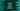# Python replace in numpy array more or less than a specific value## Python program to replace all elements of a numpy array that is more than or less than a specific value :

This post will show you how to replace all elements of a nd numpy array that is more than a value with another value. numpy provides a lot of useful methods that makes the array processing easy and quick. Even for the current problem, we have one one line solution.

## Python example program:

Let’s take a look at the python program :

``````import numpy as np

given_array = np.array([[1.2, .9, .7], [2.3, .3, 3.4], [1.1, .1, 5.5]])

print("Given array:\n{}".format(given_array))
given_array[given_array < 1.5] = 1.5

print("Modified array:\n{}".format(given_array))``````

### Explanation:

In this example program, we are creating one numpy array called given_array. We are printing the given array and in the next line, we are replacing all values in the array that are less than 1.5 with 1.5.

Finally, we are printing the same array again. It modifies the original array.

It will print the below output :

``````Given array:
[[1.2 0.9 0.7]
[2.3 0.3 3.4]
[1.1 0.1 5.5]]
Modified array:
[[1.5 1.5 1.5]
[2.3 1.5 3.4]
[1.5 1.5 5.5]]``````